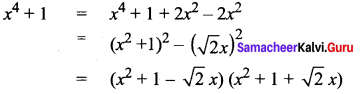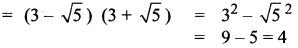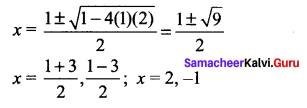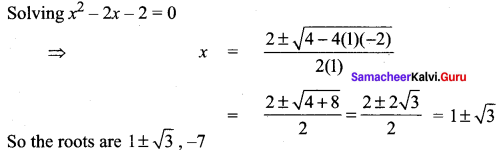## Tamilnadu Samacheer Kalvi 11th Maths Solutions Chapter 2 Basic Algebra Ex 2.7

Question 1.
Factorize: x4 + 1. (Hint: Try completing the square.)
Solution:Question 2.
If x2 + x + 1 is a factor of the polynomial 3x3 + 8x2 + 8x + a, then find the value of a.
Solution:
Let 3x3 + 8x2 + 8x + a = (x2 + x + 1) (3x + a) .
Equating coefficient of x
8 = a + 3
8 – 3 = a
a = 5

### Samacheer Kalvi 11th Maths Solutions Chapter 2 Basic Algebra Ex 2.7 Additional Questions Solved

Question 1.
Solve for x2 – 7x3 + 8x2 + 8x – 8 = 0. given 3 – $$\sqrt{5}$$ is a root
Solution:
when 3 – $$\sqrt{5}$$ is a root, 3 + $$\sqrt{5}$$ is the other root.
S.o.r. = (3 – $$\sqrt{5}$$) + (3 + $$\sqrt{5}$$) = 6The equation is x2 – 6x + 4 = 0
Now x4 – 7x3 + 8x2 + 8x – 8 = (x2 – 6x + 4) (x2 + px – 2)
Equating co-eff of x
12 + 4p = 8
4p = 8 – 12 = -4
So the other factor is x2 – x – 2
Now solving x2 – x – 2 = 0Question 2.
Solve the equation x3 + 5x2 – 16x – 14 = 0. given x + 7 is a root
Solution:
x3 + 5x2 – 16x – 14 = (x + 7) (x2 + px – 2)
Equating co-eff of x
7p – 2 = -16
7p = -16 + 2 = -14
⇒ p = -2
So the other factor is x2 – 2x – 2